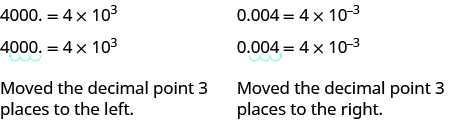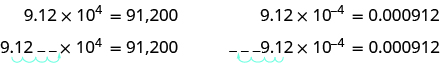## Converting Between Scientific Notation and Decimal Notation

### Learning Outcomes

• Define decimal and scientific notations
• Convert from scientific notation to decimal notation
• Convert from decimal notation to scientific notation

## Definition of Scientific Notation

Remember working with place value for whole numbers and decimals? Our number system is based on powers of $10$. We use tens, hundreds, thousands, and so on. Our decimal numbers are also based on powers of tens—tenths, hundredths, thousandths, and so on.

Consider the numbers $4000$ and $0.004$. We know that $4000$ means $4\times 1000$ and $0.004$ means $4\times {\Large\frac{1}{1000}}$. If we write the $1000$ as a power of ten in exponential form, we can rewrite these numbers in this way:

$\begin{array}{cccc}4000\hfill & & & 0.004\hfill \\ 4\times 1000\hfill & & & 4\times {\Large\frac{1}{1000}}\hfill \\ 4\times {10}^{3}\hfill & & & 4\times {\Large\frac{1}{{10}^{3}}}\hfill \\ & & & \hfill 4\times {10}^{-3}\hfill \end{array}$

When a number is written as a product of two numbers, where the first factor is a number greater than or equal to one but less than $10$, and the second factor is a power of $10$ written in exponential form, it is said to be in scientific notation.

Before we can convert between scientific and decimal notation, we need to know the difference between the two. Scientific notation is used by scientists, mathematicians, and engineers when they are working with very large or very small numbers. Using exponential notation, large and small numbers can be written in a way that is easier to read.

When a number is written in scientific notation, the exponent tells you if the term is a large or a small number. A positive exponent indicates a large number and a negative exponent indicates a small number that is between $0$ and $1$. It is difficult to understand just how big a billion or a trillion is. Here is a way to help you think about it.

 Word How many thousands Number Scientific Notation million $1000$ x $1000$ = a thousand thousands $1,000,000$ $10^6$ billion $(1000$ x $1000)$ x 1000 = a thousand millions $1,000,000,000$ $10^9$ trillion $(1000$ x $1000$ x $1000$) x $1000$ = a thousand billions $1,000,000,000,000$ $10^{12}$

$1$ billion can be written as $1,000,000,000$ or represented as $10^9$. How would $2$ billion be represented? Since $2$ billion is $2$ times $1$ billion, then $2$ billion can be written as $2\times10^9$.

A light year is the number of miles light travels in one year, about $5,880,000,000,000$.  That’s a lot of zeros, and it is easy to lose count when trying to figure out the place value of the number. Using scientific notation, the distance is $5.88\times10^{12}$ miles. The exponent of $12$ tells us how many places to count to the left of the decimal. Another example of how scientific notation can make numbers easier to read is the diameter of a hydrogen atom, which is about $0.00000005 mm$, and in scientific notation is $5\times10^{-8}$ mm. In this case the $-8$ tells us how many places to count to the right of the decimal.

Outlined in the box below are some important conventions of scientific notation format.

### Scientific Notation

A positive number is written in scientific notation if it is written as $a\times10^{n}$ where the coefficient a is $1\leq{a}<10$, and n is an integer.

It is customary in scientific notation to use $\times$ as the multiplication sign, even though we avoid using this sign elsewhere in algebra.

Look at the numbers below. Which of the numbers is written in scientific notation?

 Number Scientific Notation? Explanation $1.85\times10^{-2}$ yes $1\leq1.85<10$ $-2$ is an integer $\displaystyle 1.083\times {{10}^{\frac{1}{2}}}$ no $\displaystyle \frac{1}{2}$ is not an integer $0.82\times10^{14}$ no 0.82 is not $\geq1$ $10\times10^{3}$ no 10 is not < 10

## Convert from decimal notation to scientific notation

Now let’s compare some numbers expressed in both scientific notation and standard decimal notation in order to understand how to convert from one form to the other. Take a look at the tables below. Pay close attention to the exponent in the scientific notation and the position of the decimal point in the decimal notation.

 Large Numbers Small Numbers Decimal Notation Scientific Notation Decimal Notation Scientific Notation $500.0$ $5\times10^{2}$ $0.05$ $5\times10^{-2}$ $80,000.0$ $8\times10^{4}$ $0.0008$ $8\times10^{-4}$ $43,000,000.0$ $4.3\times10^{7}$ $0.00000043$ $4.3\times10^{-7}$ $62,500,000,000.0$ $6.25\times10^{10}$ $0.000000000625$ $6.25\times10^{-10}$

If we look at what happens to the decimal point, we can see a method to easily convert from decimal notation to scientific notation.In both cases, the decimal was moved $3$ places to get the first factor, $4$, by itself.

To write a large number in scientific notation, move the decimal point to the left to obtain a number between $1$ and $10$. Since moving the decimal point changes the value, you have to multiply the decimal by a power of $10$ so that the expression has the same value.

Let’s look at an example.

$\begin{array}{r}180,000.=18,000.0\times10^{1}\\1,800.00\times10^{2}\\180.000\times10^{3}\\18.0000\times10^{4}\\1.80000\times10^{5}\\180,000=1.8\times10^{5}\end{array}$

Notice that the decimal point was moved $5$ places to the left, and the exponent is $5$.

In the examples shown below, we will be following this general strategy for converting from decimals to scientific notation:

### Convert from decimal notation to scientific notation

1. Move the decimal point so that the first factor is greater than or equal to $1$ but less than $10$.
2. Count the number of decimal places, $n$, that the decimal point was moved.
3. Write the number as a product with a power of $10$.
• If the original number is:
• greater than $1$, the power of $10$ will be ${10}^{n}$.
• between $0$ and $1$, the power of $10$ will be ${10}^{-n}$.
4. Check.

In the examples below, we are converting large decimal values to scientific notation.

### example

Write $37,000$ in scientific notation.

Solution

 Step 1: Move the decimal point so that the first factor is greater than or equal to $1$ but less than $10$.Step 2: Count the number of decimal places, $n$ , that the decimal point was moved. $3.70000$ $4$ places Step 3: Write the number as a product with a power of $10$. $3.7\times {10}^{4}$ If the original number is: greater than 1, the power of 10 will be ${10}^{n}$ . between 0 and 1, the power of 10 will be ${10}^{\mathrm{-n}}$ Step 4: Check. ${10}^{4}$ is $10,000$ and $10,000$ times $3.7$ will be $37,000$. $37,000=3.7\times {10}^{4}$

### Example

Write the following numbers in scientific notation.

1. $920,000,000$
2. $10,200,000$
3. $100,000,000,000$

### try it

Now let’s look at converting a very small decimal number to scientific notation.  To write a small number (between $0$ and $1$) in scientific notation, you move the decimal to the right and the exponent will have to be negative, as in the following example.

$\begin{array}{r}\underset{\longrightarrow}{0.00004}=00.0004\times10^{-1}\\000.004\times10^{-2}\\0000.04\times10^{-3}\\00000.4\times10^{-4}\\000004.\times10^{-5}\\0.00004=4\times10^{-5}\end{array}$

You may notice that the decimal point was moved five places to the right until you got  to the number 4, which is between $1$ and $10�$. The exponent is $−5$.

### example

Write in scientific notation: $0.0052$

### Example

Write the following numbers in scientific notation.

1. $0.0000000000035$
2. $0.0000000102$
3. $0.00000000000000793$

### try it

In the following video you are provided with examples of how to convert both a large and a small number in decimal notation to scientific notation.

## Convert from scientific notation to decimal notation

How can we convert from scientific notation to decimal form? Let’s look at two numbers written in scientific notation and see.

$\begin{array}{cccc}9.12\times {10}^{4}\hfill & & & 9.12\times {10}^{-4}\hfill \\ 9.12\times 10,000\hfill & & & 9.12\times 0.0001\hfill \\ 91,200\hfill & & & 0.000912\hfill \end{array}$

If we look at the location of the decimal point, we can see an easy method to convert a number from scientific notation to decimal form.In both cases the decimal point moved 4 places. When the exponent was positive, the decimal moved to the right. When the exponent was negative, the decimal point moved to the left.

Recall the number of miles that light travels in a year is $5.88\times10^{12}$, and a hydrogen atom has a diameter of $5\times10^{-8}$ mm. To write each of these numbers in decimal notation, you move the decimal point the same number of places as the exponent. If the exponent is positive, move the decimal point to the right. If the exponent is negative, move the decimal point to the left.

$\begin{array}{l}5.88\times10^{12}=\underset{\longrightarrow}{5.880000000000.}=5,880,000,000,000\\5\times10^{-8}=\underset{\longleftarrow}{0.00000005.}=0.00000005\end{array}$

For each power o f$10$, you move the decimal point one place. Be careful here and don’t get carried away with the zeros—the number of zeros after the decimal point will always be $1$ less than the exponent because it takes one power of $10$ to shift that first number to the left of the decimal.

As we practice converting from scientific notation to decimal form, we will be following these steps:

### Convert scientific notation to decimal form

1. Determine the exponent, $n$, on the factor $10$.
2. Move the decimal $n$ places, adding zeros if needed.
• If the exponent is positive, move the decimal point $n$ places to the right.
• If the exponent is negative, move the decimal point $|n|$ places to the left.
3. Check.

Let’s start by converting a large number in scientific notation to decimal form.

### example

Convert to decimal form: $6.2\times {10}^{3}$

### try it

Now, let’s try converting small numbers to decimal form.

### example

Convert to decimal form: $8.9\times {10}^{-2}$

### Example

Write the following in decimal notation.

1. $4.8\times10^{-4}$
2. $3.08\times10^{6}$

To help you get a sense of the relationship between the sign of the exponent and the relative size of a number written in scientific notation, answer the following questions. You can use the textbox to wirte your ideas before you reveal the solution.

1. You are writing a number that is greater than $1$ in scientific notation.  Will your exponent be positive or negative?

2. You are writing a number that is between $0$ and $1$ in scientific notation.  Will your exponent be positive or negative?

3. What power do you need to put on $10$ to get a result of $1$?

In the next video you will see how to convert a number written in scientific notation into decimal notation.

### Summary

Large and small numbers can be written in scientific notation to make them easier to understand. In the next section, you will see that performing mathematical operations such as multiplication and division on large and small numbers is made easier by scientific notation and the rules of exponents.

## Contribute!

Did you have an idea for improving this content? We’d love your input.